Скачать презентацию Machine Learning Sudeshna Sarkar IIT Kharagpur Oct 17

• Количество слайдов: 36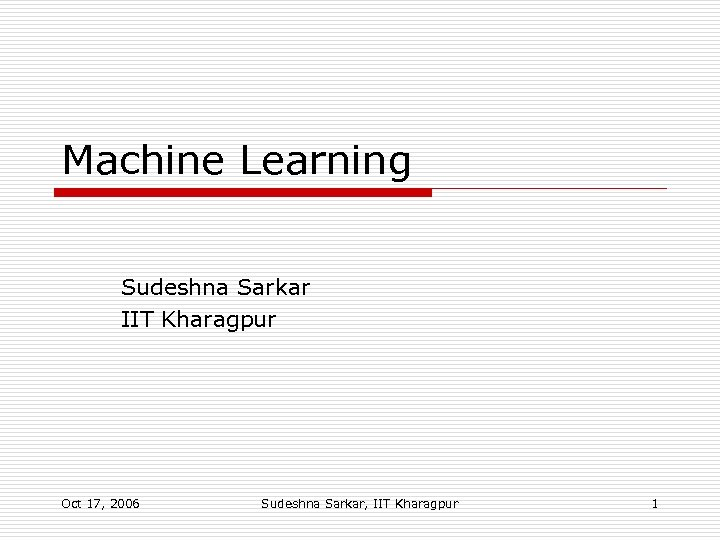Machine Learning Sudeshna Sarkar IIT Kharagpur Oct 17, 2006 Sudeshna Sarkar, IIT Kharagpur 1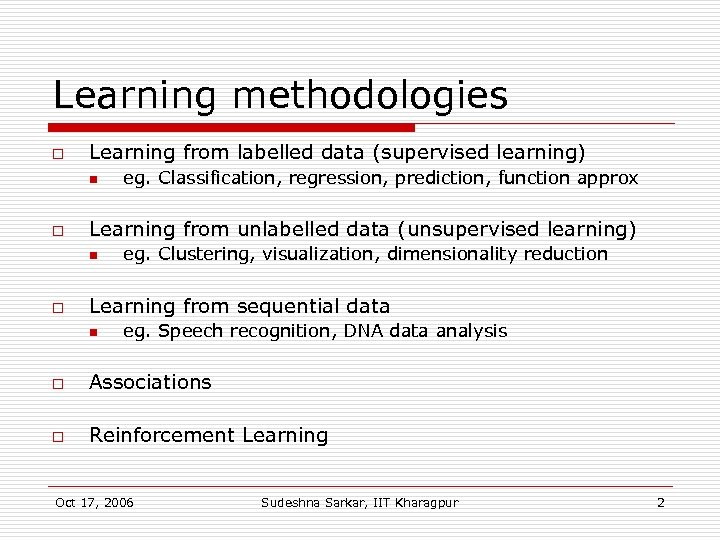Learning methodologies o Learning from labelled data (supervised learning) n o Learning from unlabelled data (unsupervised learning) n o eg. Classification, regression, prediction, function approx eg. Clustering, visualization, dimensionality reduction Learning from sequential data n eg. Speech recognition, DNA data analysis o Associations o Reinforcement Learning Oct 17, 2006 Sudeshna Sarkar, IIT Kharagpur 2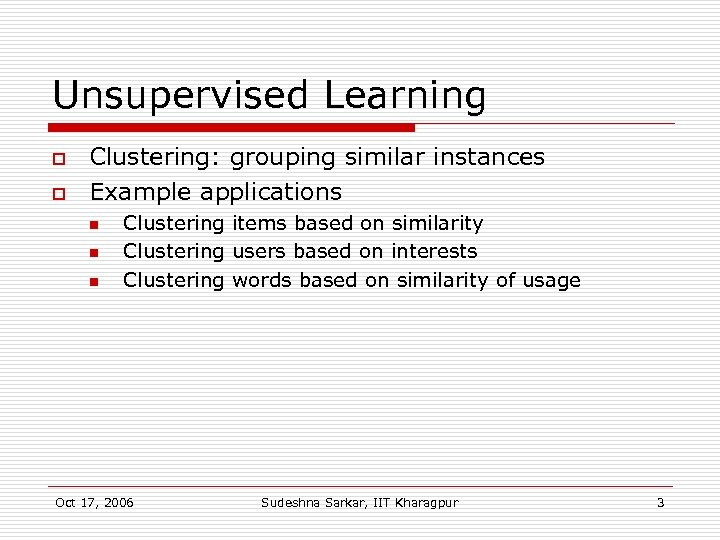Unsupervised Learning o o Clustering: grouping similar instances Example applications n n n Clustering items based on similarity Clustering users based on interests Clustering words based on similarity of usage Oct 17, 2006 Sudeshna Sarkar, IIT Kharagpur 3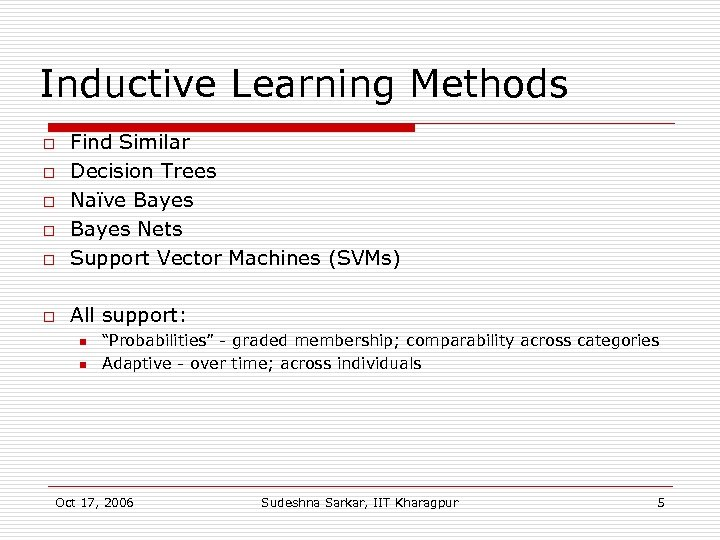Inductive Learning Methods o Find Similar Decision Trees Naïve Bayes Nets Support Vector Machines (SVMs) o All support: o o n n “Probabilities” - graded membership; comparability across categories Adaptive - over time; across individuals Oct 17, 2006 Sudeshna Sarkar, IIT Kharagpur 5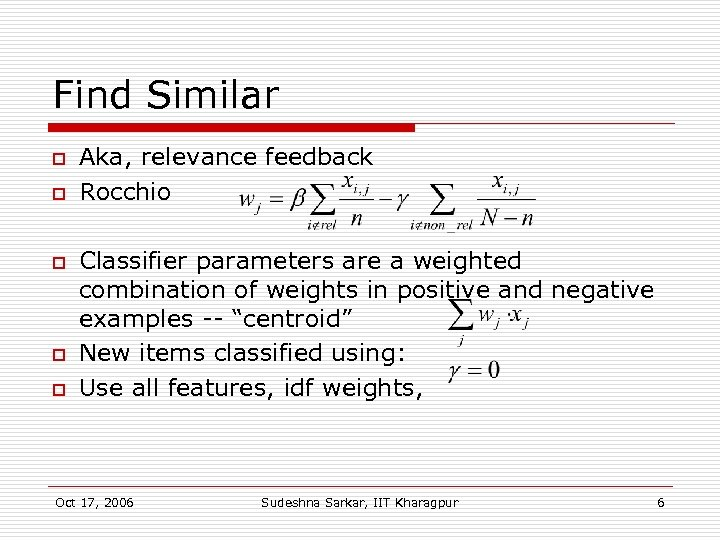Find Similar o o o Aka, relevance feedback Rocchio Classifier parameters are a weighted combination of weights in positive and negative examples -- “centroid” New items classified using: Use all features, idf weights, Oct 17, 2006 Sudeshna Sarkar, IIT Kharagpur 6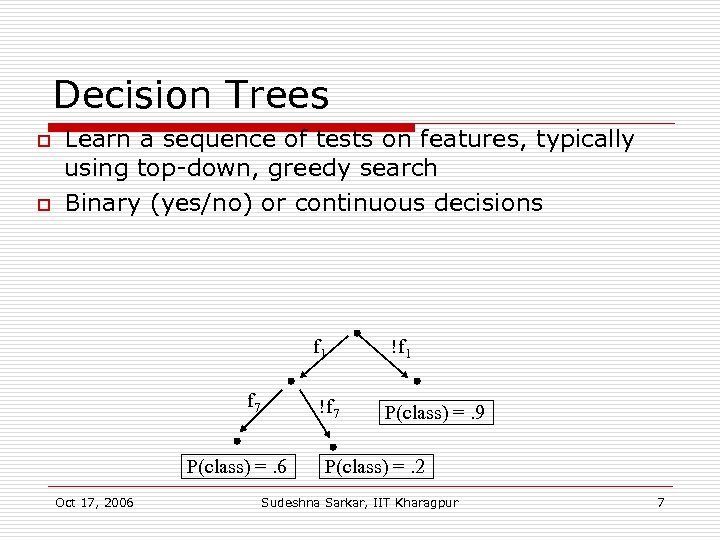Decision Trees o o Learn a sequence of tests on features, typically using top-down, greedy search Binary (yes/no) or continuous decisions f 1 !f 7 P(class) =. 6 Oct 17, 2006 !f 1 P(class) =. 9 P(class) =. 2 Sudeshna Sarkar, IIT Kharagpur 7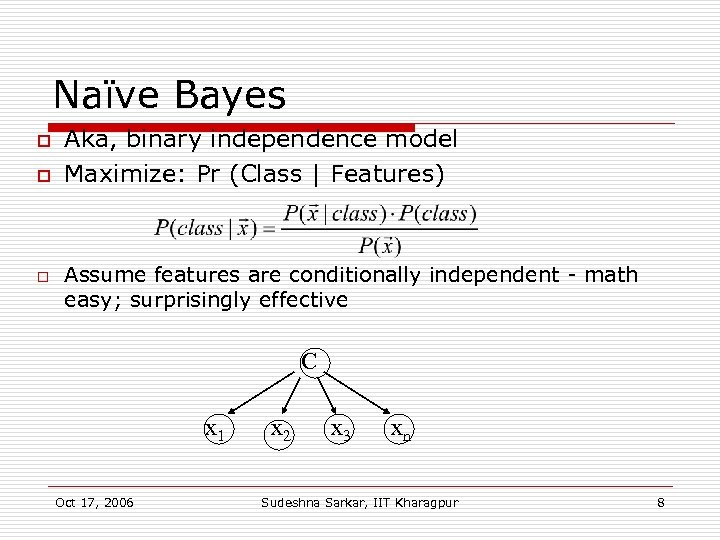Naïve Bayes o o o Aka, binary independence model Maximize: Pr (Class | Features) Assume features are conditionally independent - math easy; surprisingly effective C x 1 Oct 17, 2006 x 2 x 3 xn Sudeshna Sarkar, IIT Kharagpur 8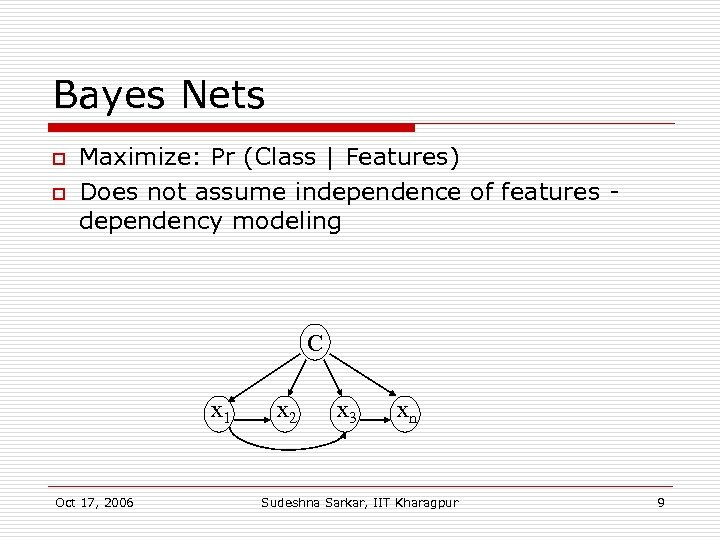Bayes Nets o o Maximize: Pr (Class | Features) Does not assume independence of features dependency modeling C x 1 Oct 17, 2006 x 2 x 3 xn Sudeshna Sarkar, IIT Kharagpur 9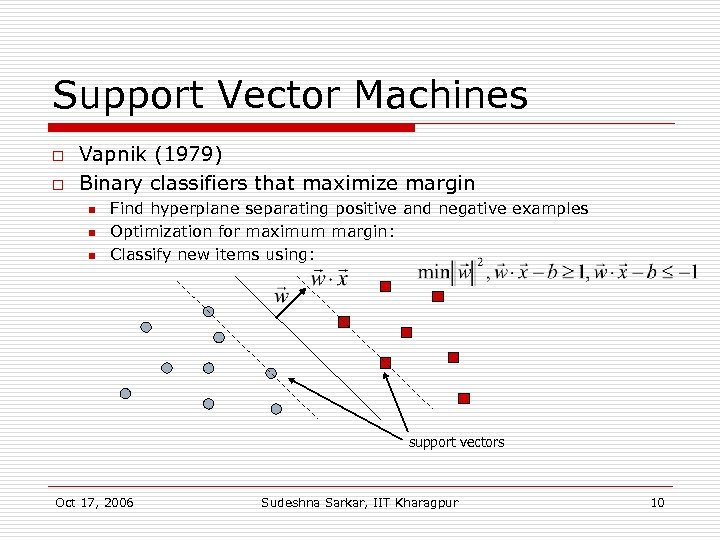Support Vector Machines o o Vapnik (1979) Binary classifiers that maximize margin n Find hyperplane separating positive and negative examples Optimization for maximum margin: Classify new items using: support vectors Oct 17, 2006 Sudeshna Sarkar, IIT Kharagpur 10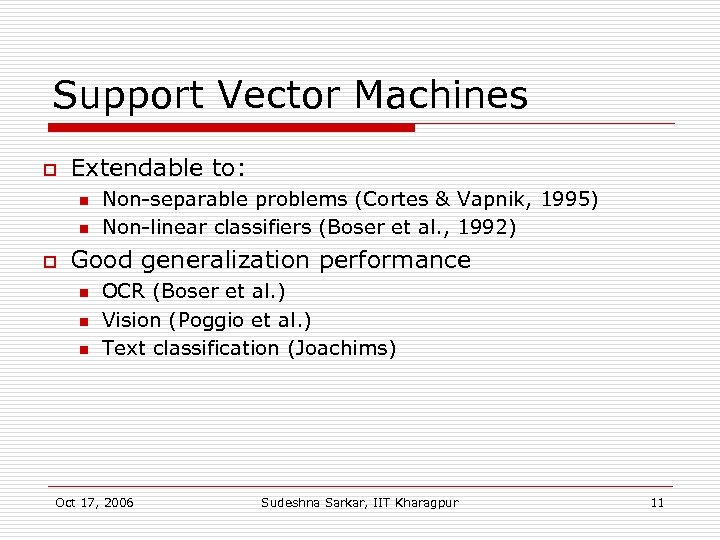Support Vector Machines o Extendable to: n n o Non-separable problems (Cortes & Vapnik, 1995) Non-linear classifiers (Boser et al. , 1992) Good generalization performance n n n OCR (Boser et al. ) Vision (Poggio et al. ) Text classification (Joachims) Oct 17, 2006 Sudeshna Sarkar, IIT Kharagpur 11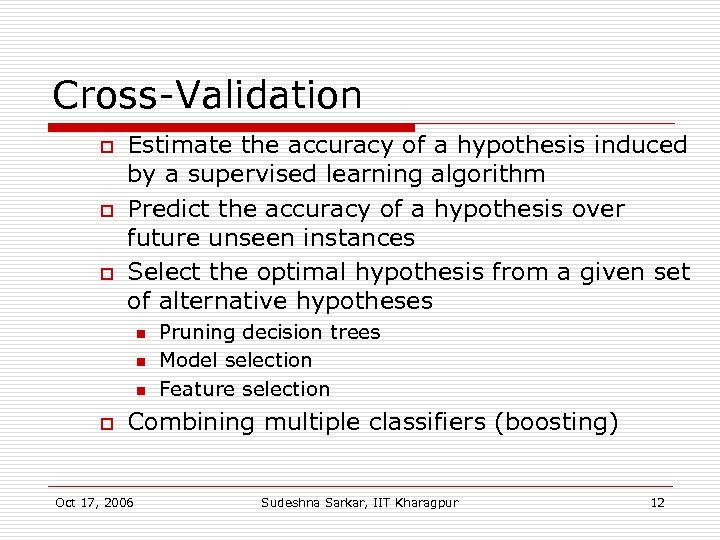Cross-Validation o o o Estimate the accuracy of a hypothesis induced by a supervised learning algorithm Predict the accuracy of a hypothesis over future unseen instances Select the optimal hypothesis from a given set of alternative hypotheses n n n o Pruning decision trees Model selection Feature selection Combining multiple classifiers (boosting) Oct 17, 2006 Sudeshna Sarkar, IIT Kharagpur 12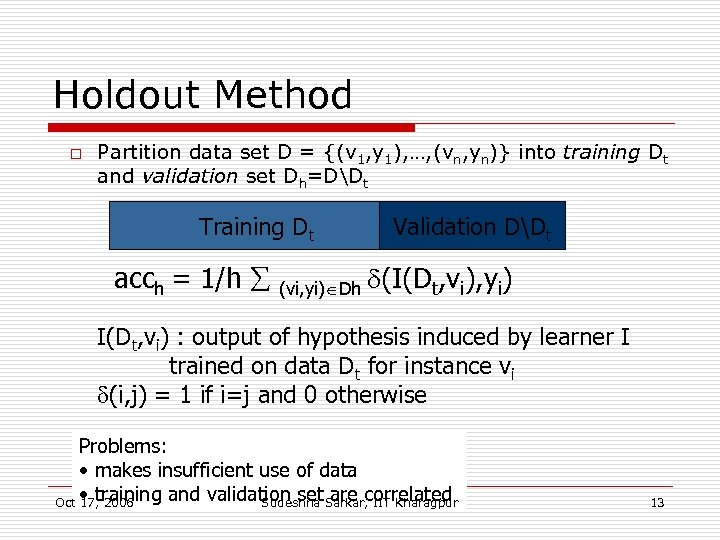Holdout Method o Partition data set D = {(v 1, y 1), …, (vn, yn)} into training Dt and validation set Dh=DDt Training Dt acch = 1/h (vi, yi) Dh Validation DDt (I(Dt, vi), yi) I(Dt, vi) : output of hypothesis induced by learner I trained on data Dt for instance vi (i, j) = 1 if i=j and 0 otherwise Problems: • makes insufficient use of data Oct • training and validation set Sarkar, IIT Kharagpur 17, 2006 Sudeshna are correlated 13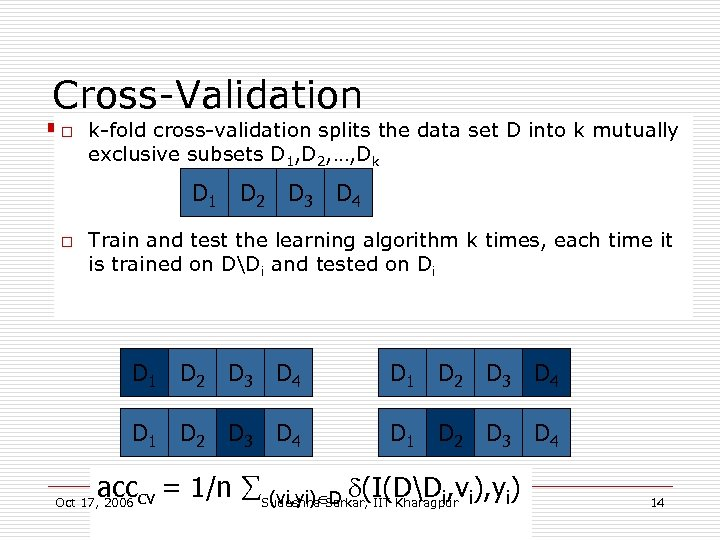Cross-Validation o k-fold cross-validation splits the data set D into k mutually exclusive subsets D 1, D 2, …, Dk D 1 D 2 D 3 D 4 o Train and test the learning algorithm k times, each time it is trained on DDi and tested on Di D 1 D 2 D 3 D 4 acccv = 1/n Sudeshna Sarkar, IIT Kharagpur i), yi) (vi, yi) D (I(DDi, v Oct 17, 2006 14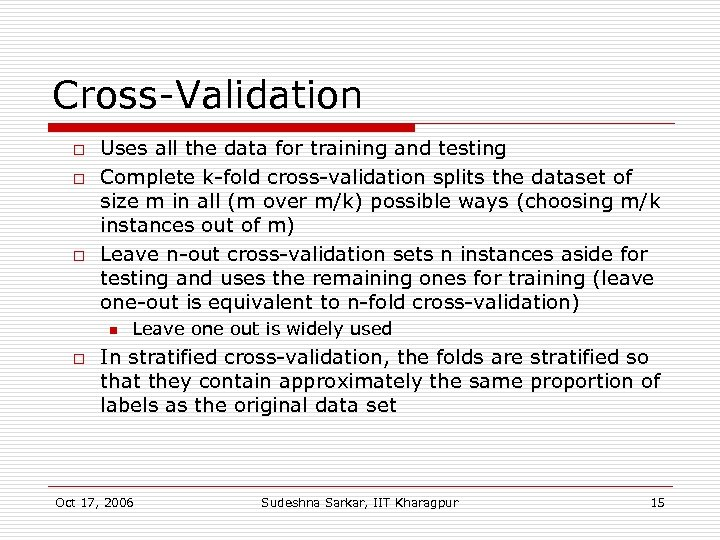Cross-Validation o o o Uses all the data for training and testing Complete k-fold cross-validation splits the dataset of size m in all (m over m/k) possible ways (choosing m/k instances out of m) Leave n-out cross-validation sets n instances aside for testing and uses the remaining ones for training (leave one-out is equivalent to n-fold cross-validation) n o Leave one out is widely used In stratified cross-validation, the folds are stratified so that they contain approximately the same proportion of labels as the original data set Oct 17, 2006 Sudeshna Sarkar, IIT Kharagpur 15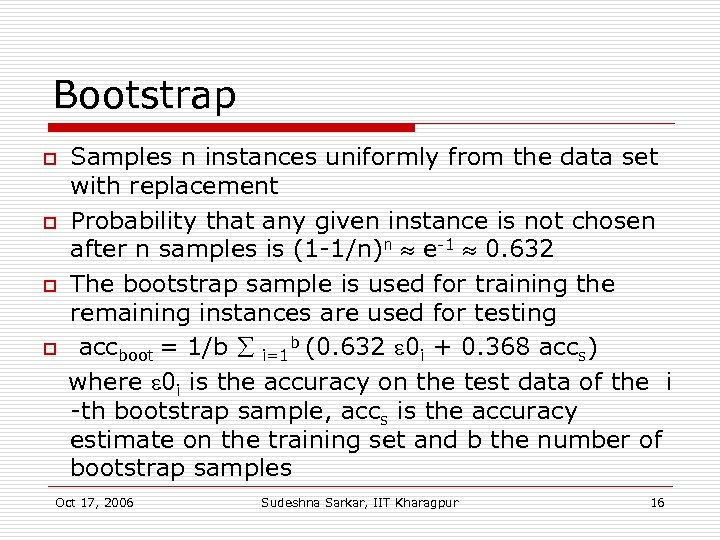Bootstrap o o Samples n instances uniformly from the data set with replacement Probability that any given instance is not chosen after n samples is (1 -1/n)n e-1 0. 632 The bootstrap sample is used for training the remaining instances are used for testing accboot = 1/b i=1 b (0. 632 0 i + 0. 368 accs) where 0 i is the accuracy on the test data of the i -th bootstrap sample, accs is the accuracy estimate on the training set and b the number of bootstrap samples Oct 17, 2006 Sudeshna Sarkar, IIT Kharagpur 16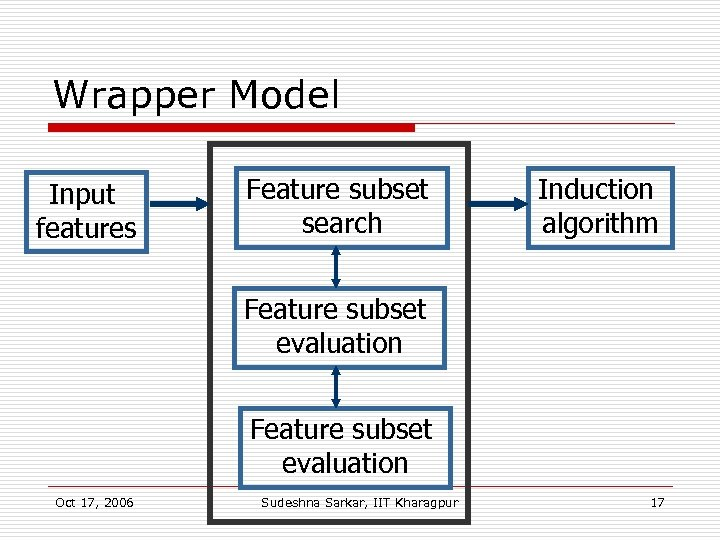Wrapper Model Input features Feature subset search Induction algorithm Feature subset evaluation Oct 17, 2006 Sudeshna Sarkar, IIT Kharagpur 17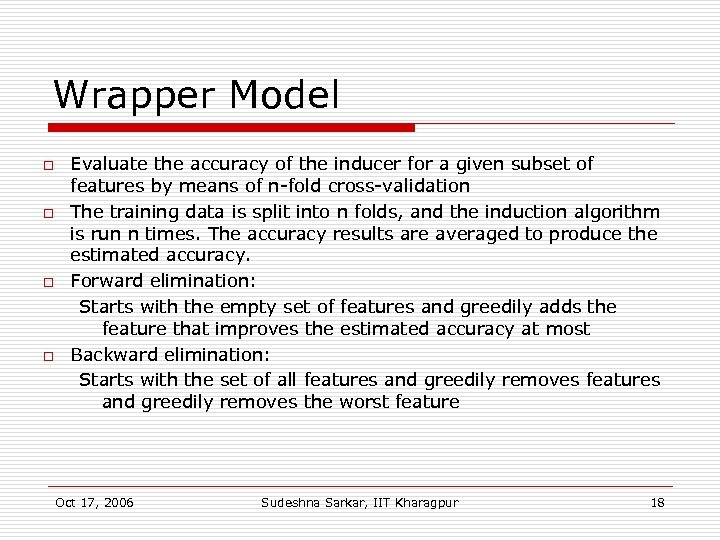Wrapper Model o o Evaluate the accuracy of the inducer for a given subset of features by means of n-fold cross-validation The training data is split into n folds, and the induction algorithm is run n times. The accuracy results are averaged to produce the estimated accuracy. Forward elimination: Starts with the empty set of features and greedily adds the feature that improves the estimated accuracy at most Backward elimination: Starts with the set of all features and greedily removes the worst feature Oct 17, 2006 Sudeshna Sarkar, IIT Kharagpur 18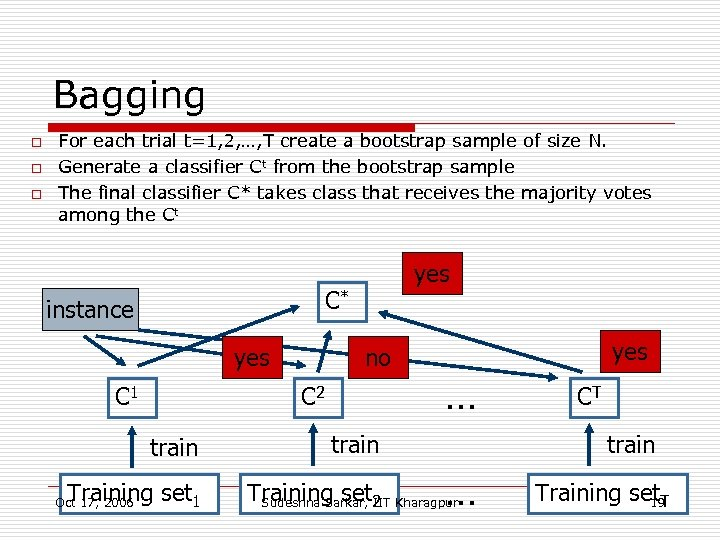Bagging o o o For each trial t=1, 2, …, T create a bootstrap sample of size N. Generate a classifier Ct from the bootstrap sample The final classifier C* takes class that receives the majority votes among the Ct C* instance yes C 1 no C 2 train Training set 1 Oct 17, 2006 yes … train CT train … Training set 2 Kharagpur Sudeshna Sarkar, IIT Training set. T 19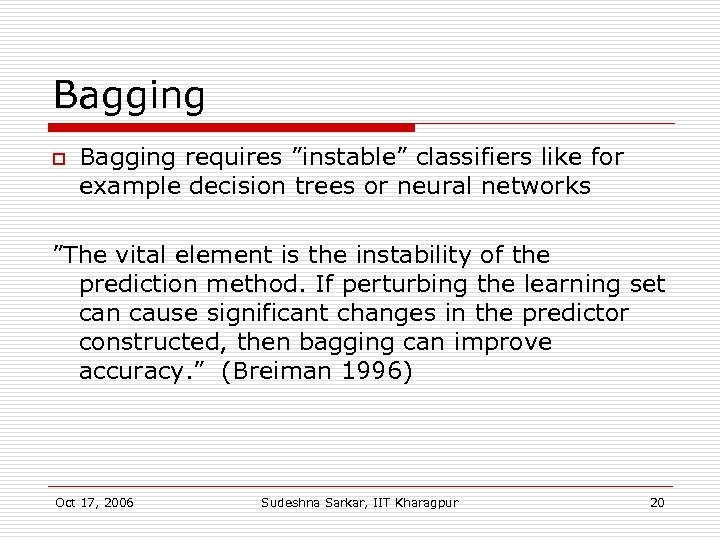Bagging o Bagging requires ”instable” classifiers like for example decision trees or neural networks ”The vital element is the instability of the prediction method. If perturbing the learning set can cause significant changes in the predictor constructed, then bagging can improve accuracy. ” (Breiman 1996) Oct 17, 2006 Sudeshna Sarkar, IIT Kharagpur 20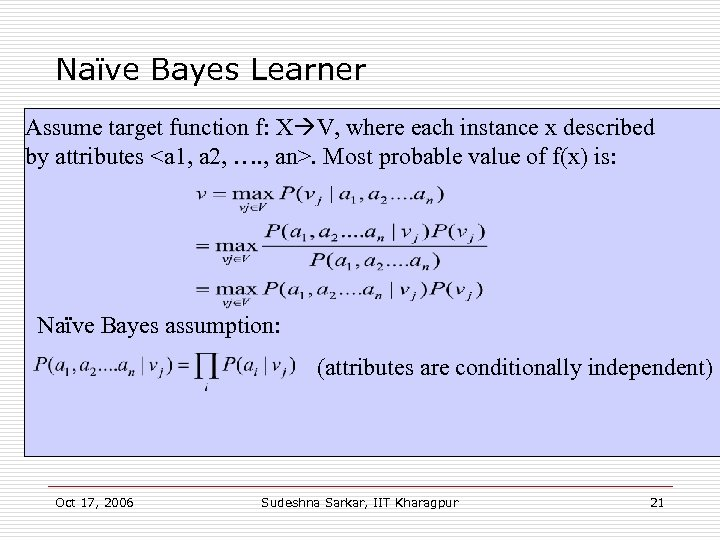Naïve Bayes Learner Assume target function f: X V, where each instance x described by attributes . Most probable value of f(x) is: Naïve Bayes assumption: (attributes are conditionally independent) Oct 17, 2006 Sudeshna Sarkar, IIT Kharagpur 21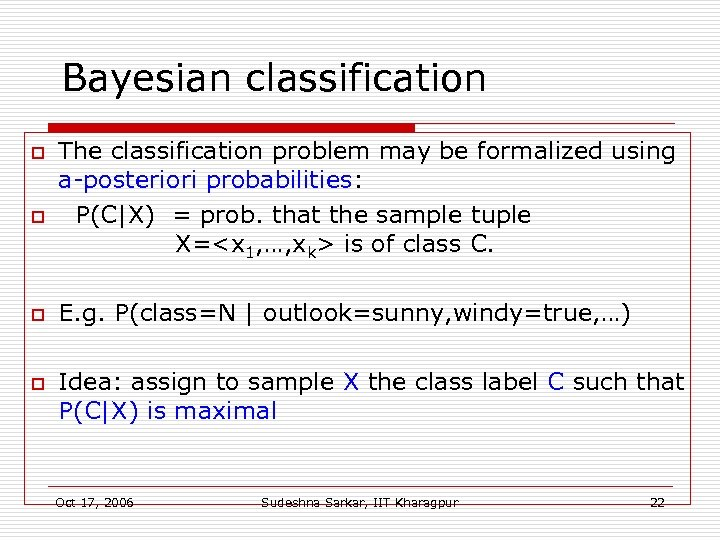Bayesian classification o o The classification problem may be formalized using a-posteriori probabilities: P(C|X) = prob. that the sample tuple X= is of class C. E. g. P(class=N | outlook=sunny, windy=true, …) Idea: assign to sample X the class label C such that P(C|X) is maximal Oct 17, 2006 Sudeshna Sarkar, IIT Kharagpur 22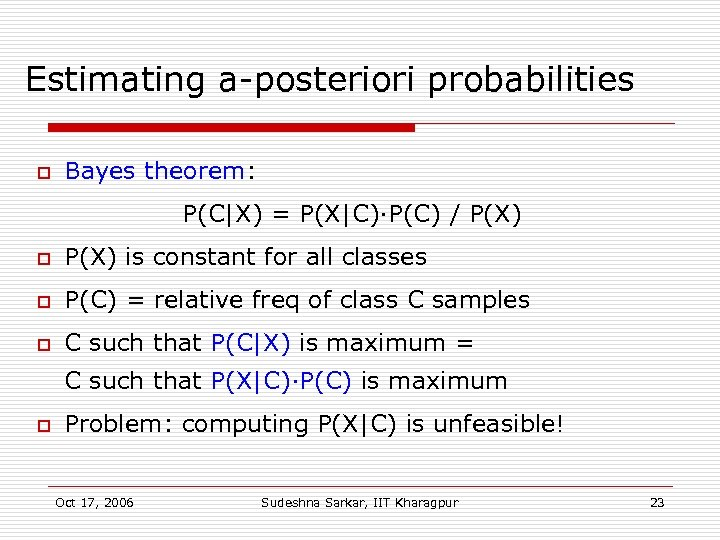Estimating a-posteriori probabilities o Bayes theorem: P(C|X) = P(X|C)·P(C) / P(X) o P(X) is constant for all classes o P(C) = relative freq of class C samples o C such that P(C|X) is maximum = C such that P(X|C)·P(C) is maximum o Problem: computing P(X|C) is unfeasible! Oct 17, 2006 Sudeshna Sarkar, IIT Kharagpur 23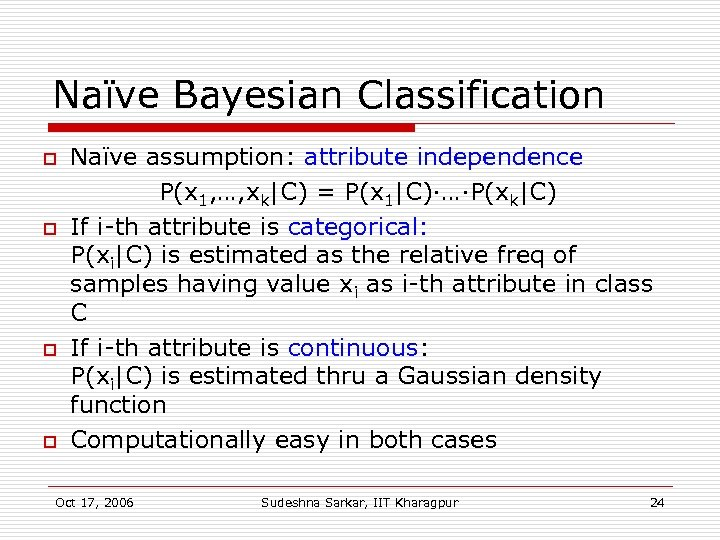Naïve Bayesian Classification o o Naïve assumption: attribute independence P(x 1, …, xk|C) = P(x 1|C)·…·P(xk|C) If i-th attribute is categorical: P(xi|C) is estimated as the relative freq of samples having value xi as i-th attribute in class C If i-th attribute is continuous: P(xi|C) is estimated thru a Gaussian density function Computationally easy in both cases Oct 17, 2006 Sudeshna Sarkar, IIT Kharagpur 24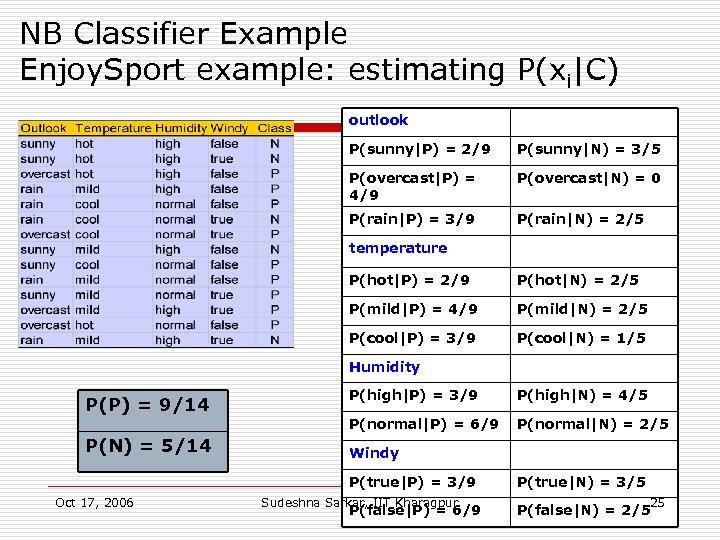NB Classifier Example Enjoy. Sport example: estimating P(xi|C) outlook P(sunny|P) = 2/9 P(sunny|N) = 3/5 P(overcast|P) = 4/9 P(overcast|N) = 0 P(rain|P) = 3/9 P(rain|N) = 2/5 temperature P(hot|P) = 2/9 P(hot|N) = 2/5 P(mild|P) = 4/9 P(mild|N) = 2/5 P(cool|P) = 3/9 P(cool|N) = 1/5 Humidity P(P) = 9/14 P(N) = 5/14 P(high|P) = 3/9 P(high|N) = 4/5 P(normal|P) = 6/9 P(normal|N) = 2/5 Windy P(true|P) = 3/9 Oct 17, 2006 Sudeshna Sarkar, IIT Kharagpur P(false|P) = 6/9 P(true|N) = 3/5 25 P(false|N) = 2/5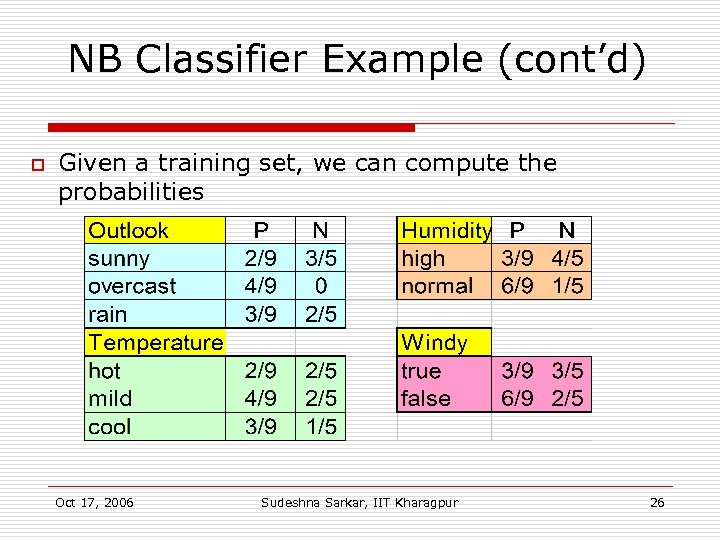NB Classifier Example (cont’d) o Given a training set, we can compute the probabilities Oct 17, 2006 Sudeshna Sarkar, IIT Kharagpur 26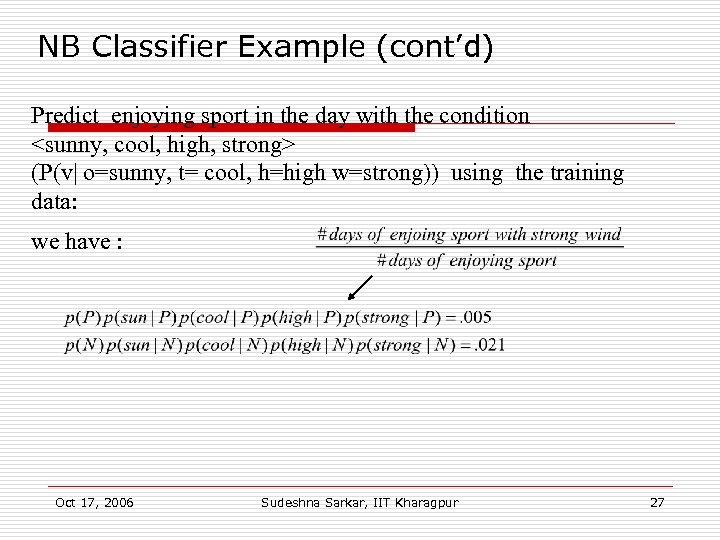NB Classifier Example (cont’d) Predict enjoying sport in the day with the condition (P(v| o=sunny, t= cool, h=high w=strong)) using the training data: we have : Oct 17, 2006 Sudeshna Sarkar, IIT Kharagpur 27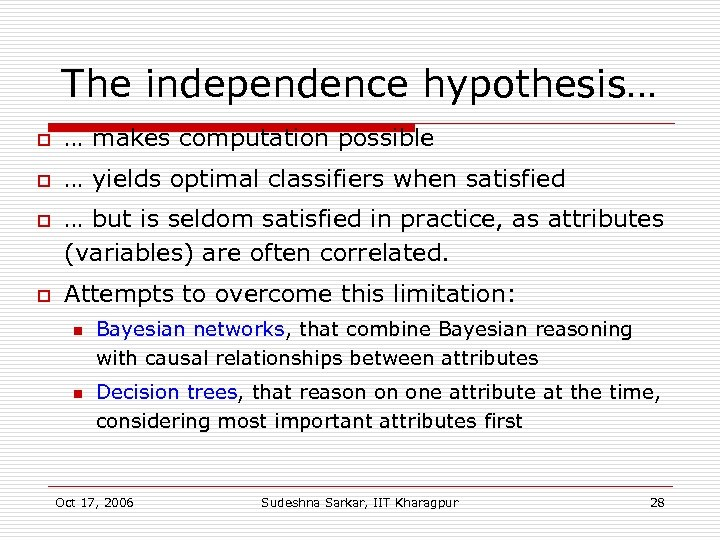The independence hypothesis… o … makes computation possible o … yields optimal classifiers when satisfied o o … but is seldom satisfied in practice, as attributes (variables) are often correlated. Attempts to overcome this limitation: n n Bayesian networks, that combine Bayesian reasoning with causal relationships between attributes Decision trees, that reason on one attribute at the time, considering most important attributes first Oct 17, 2006 Sudeshna Sarkar, IIT Kharagpur 28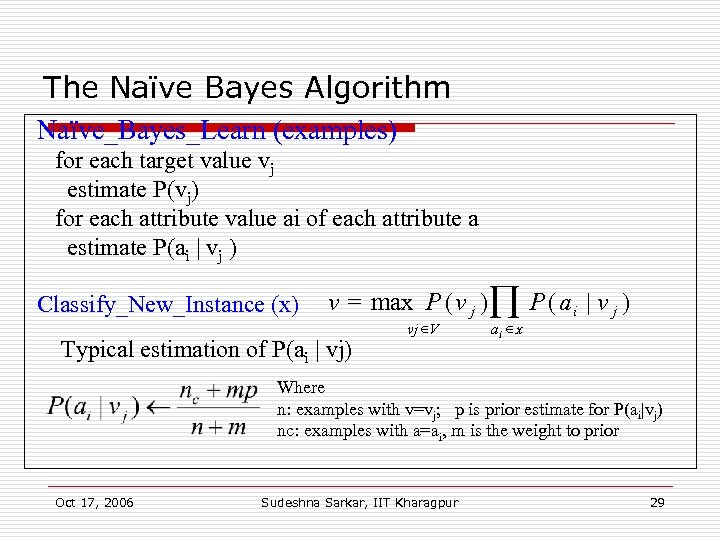The Naïve Bayes Algorithm Naïve_Bayes_Learn (examples) for each target value vj estimate P(vj) for each attribute value ai of each attribute a estimate P(ai | vj ) Classify_New_Instance (x) v = max P ( v j ) Õ P ( a i | v j ) Typical estimation of P(ai | vj) vj V ai x Where n: examples with v=vj; p is prior estimate for P(ai|vj) nc: examples with a=ai, m is the weight to prior Oct 17, 2006 Sudeshna Sarkar, IIT Kharagpur 29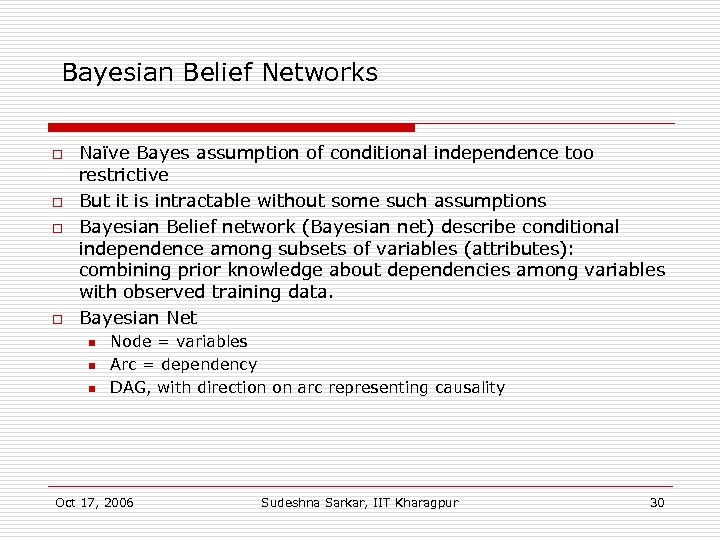Bayesian Belief Networks o o Naïve Bayes assumption of conditional independence too restrictive But it is intractable without some such assumptions Bayesian Belief network (Bayesian net) describe conditional independence among subsets of variables (attributes): combining prior knowledge about dependencies among variables with observed training data. Bayesian Net n n n Node = variables Arc = dependency DAG, with direction on arc representing causality Oct 17, 2006 Sudeshna Sarkar, IIT Kharagpur 30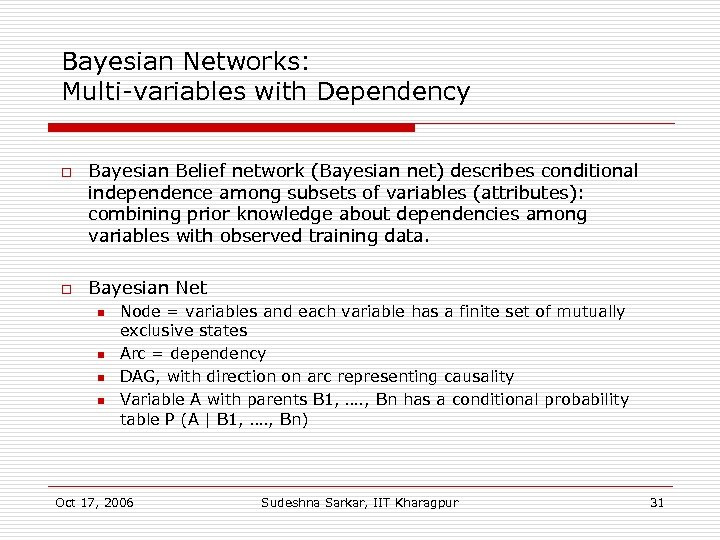Bayesian Networks: Multi-variables with Dependency o o Bayesian Belief network (Bayesian net) describes conditional independence among subsets of variables (attributes): combining prior knowledge about dependencies among variables with observed training data. Bayesian Net n n Node = variables and each variable has a finite set of mutually exclusive states Arc = dependency DAG, with direction on arc representing causality Variable A with parents B 1, …. , Bn has a conditional probability table P (A | B 1, …. , Bn) Oct 17, 2006 Sudeshna Sarkar, IIT Kharagpur 31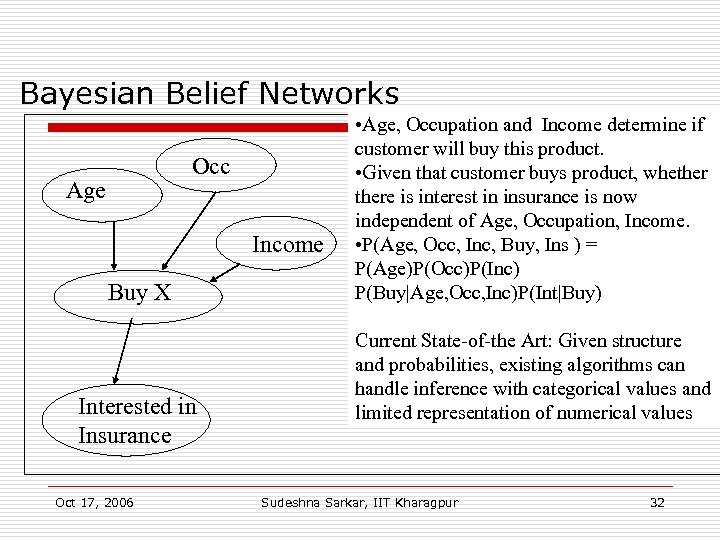Bayesian Belief Networks Occ Age Income Buy X Interested in Insurance Oct 17, 2006 • Age, Occupation and Income determine if customer will buy this product. • Given that customer buys product, whethere is interest in insurance is now independent of Age, Occupation, Income. • P(Age, Occ, Inc, Buy, Ins ) = P(Age)P(Occ)P(Inc) P(Buy|Age, Occ, Inc)P(Int|Buy) Current State-of-the Art: Given structure and probabilities, existing algorithms can handle inference with categorical values and limited representation of numerical values Sudeshna Sarkar, IIT Kharagpur 32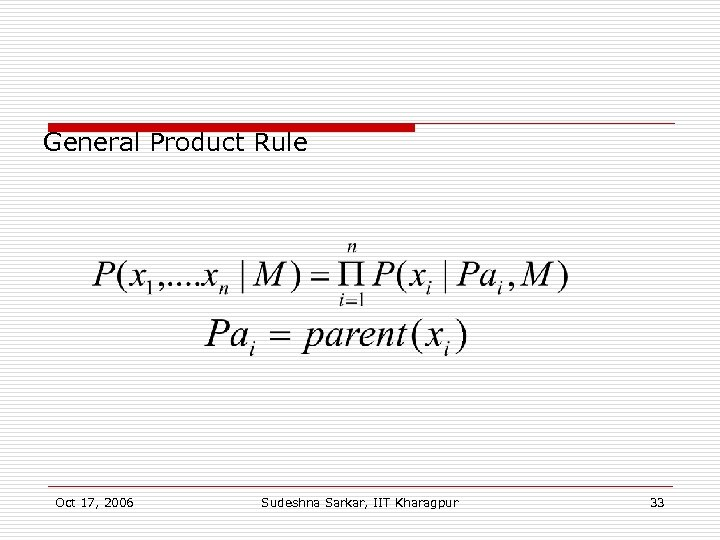General Product Rule Oct 17, 2006 Sudeshna Sarkar, IIT Kharagpur 33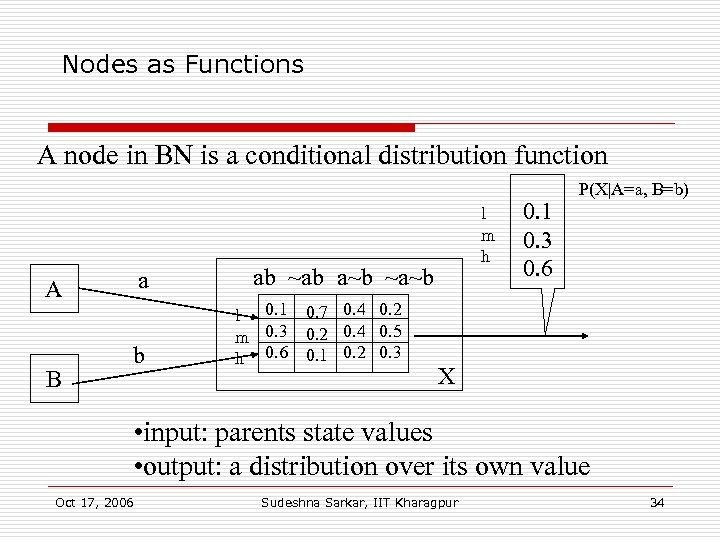Nodes as Functions A node in BN is a conditional distribution function a A B b l m h ab ~ab a~b ~a~b l 0. 1 m 0. 3 h 0. 6 0. 7 0. 4 0. 2 0. 4 0. 5 0. 1 0. 2 0. 3 0. 1 0. 3 0. 6 P(X|A=a, B=b) X • input: parents state values • output: a distribution over its own value Oct 17, 2006 Sudeshna Sarkar, IIT Kharagpur 34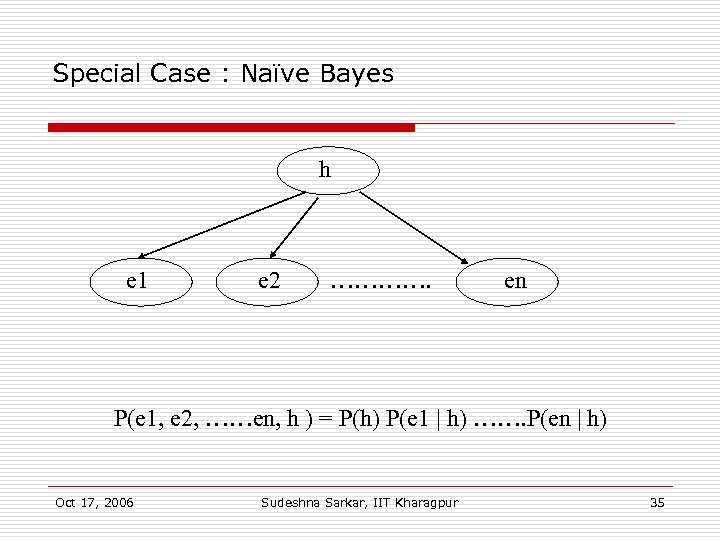Special Case : Naïve Bayes h e 1 e 2 …………. en P(e 1, e 2, ……en, h ) = P(h) P(e 1 | h) ……. P(en | h) Oct 17, 2006 Sudeshna Sarkar, IIT Kharagpur 35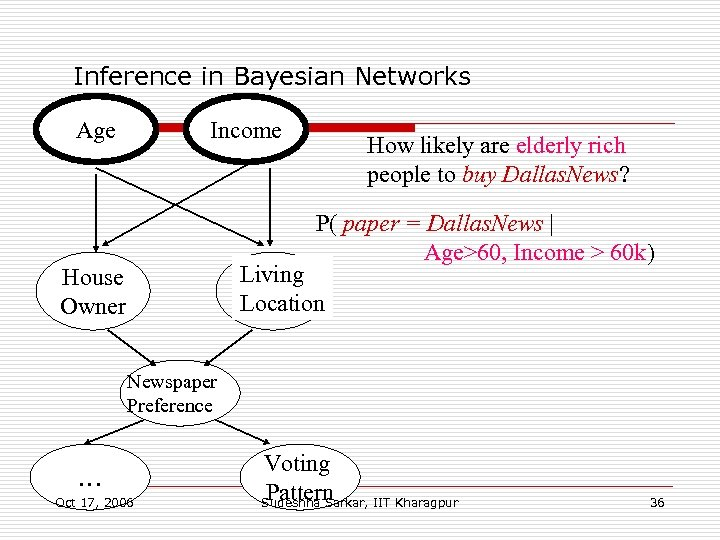Inference in Bayesian Networks Age Income How likely are elderly rich people to buy Dallas. News? P( paper = Dallas. News | Age>60, Income > 60 k) Living Location House Owner Newspaper Preference … Oct 17, 2006 Voting Pattern Sudeshna Sarkar, IIT Kharagpur 36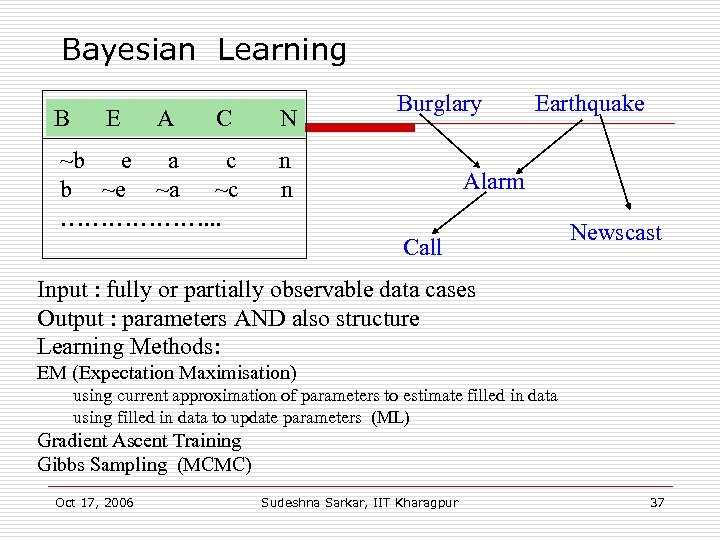Bayesian Learning B E A C N ~b e a c b ~e ~a ~c ………………. . . Burglary n n Earthquake Alarm Call Newscast Input : fully or partially observable data cases Output : parameters AND also structure Learning Methods: EM (Expectation Maximisation) using current approximation of parameters to estimate filled in data using filled in data to update parameters (ML) Gradient Ascent Training Gibbs Sampling (MCMC) Oct 17, 2006 Sudeshna Sarkar, IIT Kharagpur 37